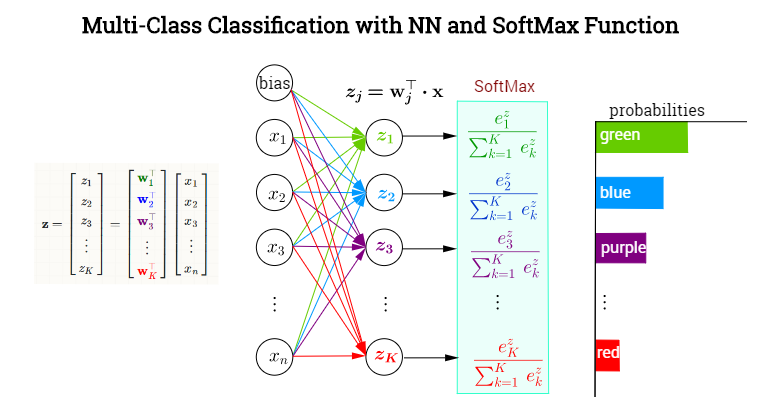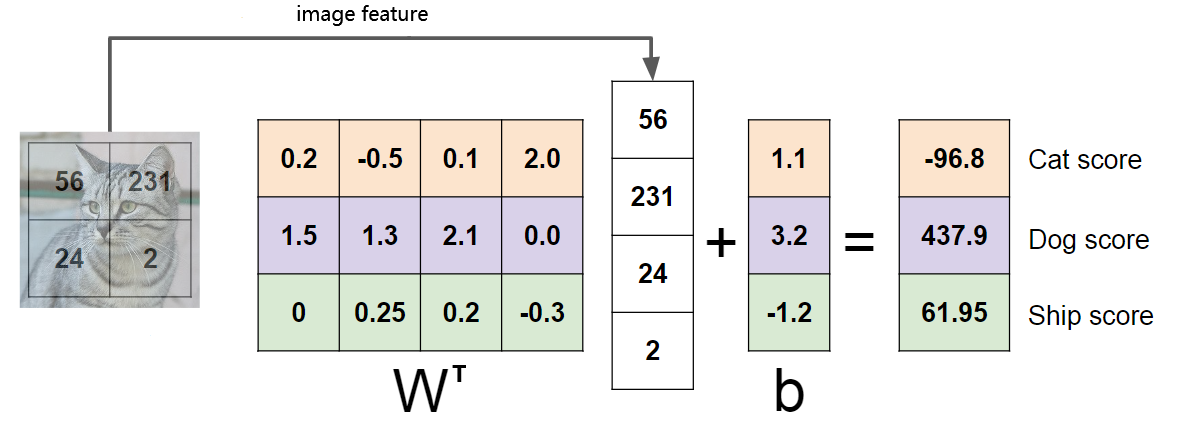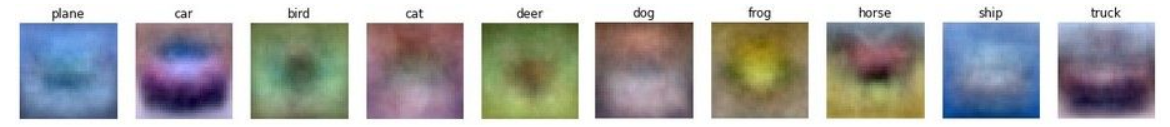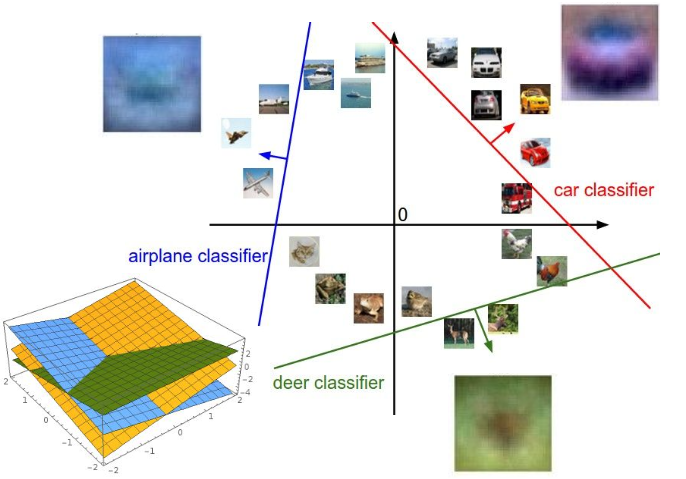# 全连接层与Softmax回顾$\hat{\mathrm{y}} = softmax(\mathrm{z}) = softmax(\mathrm{W}^{T} \mathrm{x} + \mathrm{b})$

$softmax(z_j) = \frac{e^{z_j}}{\sum_K e^{z_j}}$

$\hat{y_j} = softmax(z_j) = softmax(\mathrm{w}_{j} \cdot \mathrm{x} + b_j)$

# 加权角度

$z_j = \mathrm{w}_{j} \cdot \mathrm{x} + b_j = w_{j1} x_1 + w_{j2} x_2 + \dots + w_{jn} x_n + b_j$

$\mathrm{w}_{j}$视为第$j$类下特征的权重，即每维特征的重要程度、对最终分数的影响程度，通过对特征加权求和得到每个类别的分数，再经过Softmax映射为概率。

# 模板匹配# 几何角度

$d_j = \frac{\mathrm{w}_{j} \cdot \mathrm{x} + b_j}{||\mathrm{w}_{j}||}$# Softmax的作用

$\hat{y_j} = softmax(z_j) = \frac{e^{z_j}}{\sum_K e^{z_j}}$

# 总结

• 加权角度，将权重视为每维特征的重要程度，可以帮助理解L1、L2等正则项
• 模板匹配角度，可以帮助理解参数的可视化
• 几何角度，将特征视为多维空间中的点，可以帮助理解一些损失函数背后的设计思想（希望不同类的点具有何种性质）